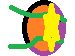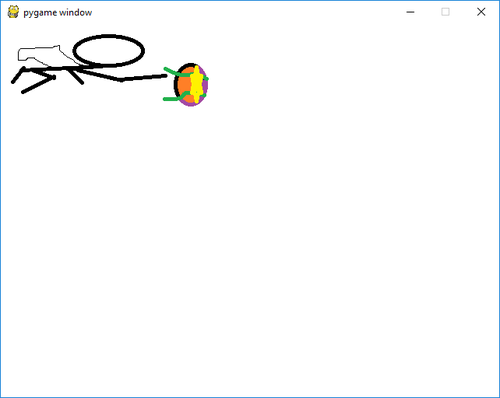# Game Engine development: Pygame - Part 3

Posted on Nov. 21, 2018

Last time, we made a simple game where we can move our hero character. But the game world is currently barren. Let's change this by having our avatar shoot some attacks.

Behold my paint drawing of an attack!Yes, it is still badly drawn, but you're not here to become an artist. You're here to learn to how make games.

## Pew pew

What we are going to do is spawn our shot when the player presses the spacebar button. Here is the code for that:

``````import pygame

resolution = (640, 480)
isRunning = True

pygame.init()
screen = pygame.display.set_mode(resolution)
clock = pygame.time.Clock()

hero = pygame.image.load("hero.png").convert()
hero.set_colorkey((255, 128, 128))
hero_pos = [0, 0]

shot = pygame.image.load("shot.png").convert()
shot.set_colorkey((255, 128, 128))
shot_pos = [-100, -100]

while isRunning:
screen.fill((255, 255, 255))
tick = clock.tick(60)

for event in pygame.event.get():
if event.type == pygame.KEYDOWN:
if event.key == pygame.K_ESCAPE:
isRunning = False

if event.type == pygame.QUIT:
isRunning  = False

keystate = pygame.key.get_pressed()
if keystate[pygame.K_LEFT]:
hero_pos -= 10
if keystate[pygame.K_RIGHT]:
hero_pos += 10
if keystate[pygame.K_UP]:
hero_pos -= 10
if keystate[pygame.K_DOWN]:
hero_pos += 10
if keystate[pygame.K_SPACE]:
shot_pos = hero_pos[:]
shot_pos += 200
shot_pos += 50

screen.blit(hero, hero_pos)
screen.blit(shot, shot_pos)

pygame.display.flip()

pygame.quit()
``````

By default, we hide our shot outside our game area (shot_pos = [-100, -100]). We can put a boolean flag to not show the sprite, or do what some games do: hide their game objects somewhere in the game world.

Adding a hidden flag will be easy once we refactor this into a game object. But I want to show an alternative approach of hiding objects in-game.

When the spacebar is pushed, we move the shot to where the hero is. Take not that in Python, objects are passed by reference. hero_pos[:] means we are making a copy of a list (alternatively, we could write this as list(hero_pos). Both are practically the same). If we don't copy the list, once we update shot_pos, we will also change hero_pos (give it a try by removing [:] in the code).

We add some offset values so that our shot will spawn a little bit ahead of our sprite. Finally, we blit the shot.

Let's see what it looks like:Looking good. But let's try moving while shooting.

Huh. This is strange. Or not strange if you realize it: we only have ONE shot. We're not actually spawning a shot, we're just moving that one shot around.

When making a game, we need the ability to spawn a new game object using code. In object oriented programming terms, this is instantiating a new object. It's now time to refactor our shot into a game object.

## Game Objects

How do we make it so that new game objects are spawned in game? The naive way would be to make multiple variables for our shot (shot, shot_pos, shot1, shot1_pos, shot2, shot2_pos, ...). This would get unwieldy very quick.

``````shot1 = pygame.image.load("shot.png").convert()
shot1.set_colorkey((255, 128, 128))
shot1_pos = [-100, -100]

shot2 = pygame.image.load("shot.png").convert()
shot2.set_colorkey((255, 128, 128))
shot2_pos = [-100, -100]

shot3 = pygame.image.load("shot.png").convert()
shot3.set_colorkey((255, 128, 128))
shot3_pos = [-100, -100]
``````

This is where the concept of OOP becomes useful. Most students are taught the classic car, vehicle, truck classes (or something similar). I find when teaching OOP, it becomes intuitive to the students once I show them the above problem (multiple code and variable for basically the same concept) and the OOP solution.

Speaking of which, here's our code with the game object:

``````# main.py
import pygame

class Shot:
def __init__(self, pos):
self.image = pygame.image.load("shot.png").convert()
self.image.set_colorkey((255, 128, 128))

pos = pos[:]
pos += 200
pos += 50

self.pos = pos

def draw(self, screen):
screen.blit(self.image, self.pos)

resolution = (640, 480)
isRunning = True

pygame.init()
screen = pygame.display.set_mode(resolution)
clock = pygame.time.Clock()

hero = pygame.image.load("hero.png").convert()
hero.set_colorkey((255, 128, 128))
hero_pos = [0, 0]

shots = []

while isRunning:
screen.fill((255, 255, 255))
tick = clock.tick(60)

for event in pygame.event.get():
if event.type == pygame.KEYDOWN:
if event.key == pygame.K_ESCAPE:
isRunning = False

if event.type == pygame.QUIT:
isRunning  = False

keystate = pygame.key.get_pressed()
if keystate[pygame.K_LEFT]:
hero_pos -= 10
if keystate[pygame.K_RIGHT]:
hero_pos += 10
if keystate[pygame.K_UP]:
hero_pos -= 10
if keystate[pygame.K_DOWN]:
hero_pos += 10
if keystate[pygame.K_SPACE]:
new_shot = Shot(hero_pos)
shots.append(new_shot)

screen.blit(hero, hero_pos)

for shot in shots:
shot.draw(screen)

pygame.display.flip()

pygame.quit()
``````

Play around with the above. You should see something like this:

Hey would you look at that, we're actually spawning new game objects now. You can play with this using the commit found here.

## Refactoring

Before we continue with the tutorial, its time for us to refactor our code. The code for the previous sections used to fit in under one page. But if we keep everything in one file, it would be hard to track the changes (especially for those following along and not looking at my commits). Going forward, I'll mark the name of the file with a comment.

``````# gameobject.py
import pygame

class Hero:
def __init__(self, pos):
self.image = pygame.image.load("hero.png").convert()
self.image.set_colorkey((255, 128, 128))

self.pos = pos[:]

def draw(self, screen):
screen.blit(self.image, self.pos)

class Shot:
def __init__(self, pos):
self.image = pygame.image.load("shot.png").convert()
self.image.set_colorkey((255, 128, 128))

pos = pos[:]
pos += 200
pos += 50

self.pos = pos

def draw(self, screen):
screen.blit(self.image, self.pos)
``````

``````# main.py
import pygame
from gameobject import Shot, Hero

resolution = (640, 480)
isRunning = True

pygame.init()
screen = pygame.display.set_mode(resolution)
clock = pygame.time.Clock()

hero = Hero([0, 0])
shots = []

while isRunning:
screen.fill((255, 255, 255))
tick = clock.tick(60)

for event in pygame.event.get():
if event.type == pygame.KEYDOWN:
if event.key == pygame.K_ESCAPE:
isRunning = False

if event.type == pygame.QUIT:
isRunning  = False

keystate = pygame.key.get_pressed()
if keystate[pygame.K_LEFT]:
hero.pos -= 10
if keystate[pygame.K_RIGHT]:
hero.pos += 10
if keystate[pygame.K_UP]:
hero.pos -= 10
if keystate[pygame.K_DOWN]:
hero.pos += 10
if keystate[pygame.K_SPACE]:
new_shot = Shot(hero.pos)
shots.append(new_shot)

hero.draw(screen)

for shot in shots:
shot.draw(screen)

pygame.display.flip()

pygame.quit()
``````

One rule in refactoring is to focus on changing one thing first and then testing. In our case, we refactored out the shot class from main.py into its own file. After testing, I went ahead and change Hero into a game object.

Programming tip

When refactoring, it is highly recommended you have automated tests. Making an automated test is beyond the scope of this tutorial. And its even possible to unit test games.

To simplify this tutorial, I will skip automated tests. It also help that this code have a final form that I have in mind. So I know what changes refactoring will do.

tl;dr: Automated unit tests helps in refactoring.

Our next step in refactoring is to simplify our game object classes. Notice that we've repeated some code twice (once in Hero and again in Shot). OOP allows us to minimize repeated code between two classes. This is the reason why class inheritance is helpful in development - you only need to code once.

``````# gameobject.py
import pygame

class GameObject:
def __init__(self, filename, pos):
self.image = pygame.image.load(filename).convert()
self.image.set_colorkey((255, 128, 128))

self.pos = pos[:]

def draw(self, screen):
screen.blit(self.image, self.pos)

class Hero(GameObject):
def __init__(self, pos):
super().__init__("hero.png", pos)

class Shot(GameObject):
def __init__(self, pos):
super().__init__("shot.png", pos)
self.pos += 200
self.pos += 50
``````

Look at that! This gameobject.py is shorter than the previous one and it's logic is exactly the same. If you never paid attention in programming class, this is why OOP is useful in programming!

One last refactoring is needed: our hero's movement is inside the game loop. While there's nothing wrong with this, as our game logic becomes complicated (like for example, we want to have different characters with different speed), it'll be hard to find the game rules for a game object.

There are many ways of organizing game logic code such as event systems and component based game objects. We will be exploring these in a latter chapter of this game engine programming series.

But for now, for simplicity, let's go for a straightforward approach: game logic that affects a game object should be inside the game object class, and game logic that affects the game world should on the game loop.

``````# main.py
import pygame
from gameobject import Shot, Hero

resolution = (640, 480)
isRunning = True

pygame.init()
screen = pygame.display.set_mode(resolution)
clock = pygame.time.Clock()

hero = Hero([0, 0])
shots = []

while isRunning:
screen.fill((255, 255, 255))
tick = clock.tick(60)

for event in pygame.event.get():
if event.type == pygame.KEYDOWN:
if event.key == pygame.K_ESCAPE:
isRunning = False

if event.type == pygame.QUIT:
isRunning  = False

keystate = pygame.key.get_pressed()
if keystate[pygame.K_SPACE]:
new_shot = Shot(hero.pos)
shots.append(new_shot)

hero.update()
for shot in shots:
shot.update()

hero.draw(screen)
for shot in shots:
shot.draw(screen)

pygame.display.flip()

pygame.quit()
``````

``````# gameobject.py
import pygame

class GameObject:
def __init__(self, filename, pos):
self.image = pygame.image.load(filename).convert()
self.image.set_colorkey((255, 128, 128))

self.pos = pos[:]

def draw(self, screen):
screen.blit(self.image, self.pos)

def update(self):
pass

class Hero(GameObject):
def __init__(self, pos):
super().__init__("hero.png", pos)
self.speed = 10

def update(self):
keystate = pygame.key.get_pressed()

if keystate[pygame.K_LEFT]:
self.pos -= self.speed
if keystate[pygame.K_RIGHT]:
self.pos += self.speed
if keystate[pygame.K_UP]:
self.pos -= self.speed
if keystate[pygame.K_DOWN]:
self.pos += self.speed

class Shot(GameObject):
def __init__(self, pos):
super().__init__("shot.png", pos)
self.pos += 200
self.pos += 50
``````

I've also removed the hardcoded speed value and moved it to the constructor. This way, it'll be easy for us to tweak the player's movement speed later.

For those who want to follow along, you can see what our directory looks like here.

## Tim tim tim

Let's get back to a problem in our game logic: our shots are boring! They just stand there. They do nothing!

Let's add some animation:

``````# gameobject.py
[...]
class Shot(GameObject):
def __init__(self, pos):
super().__init__("shot.png", pos)
self.pos += 200
self.pos += 50

self.speed = 1

def update(self):
self.pos += self.speed
``````

Well, its a cute attack, but we can do better. Let's boost the speed to 100!

``````# gameobject.py
[...]
class Shot(GameObject):
def __init__(self, pos):
super().__init__("shot.png", pos)
self.pos += 200
self.pos += 50

self.speed = 100

def update(self):
self.pos += self.speed
``````

Now that's too extreme. You know what? Variety is the spice of life. So we'll let the RNG (Random Number God) handle this.

``````# gameobject.py
import random
[...]
class Shot(GameObject):
def __init__(self, pos):
super().__init__("shot.png", pos)
self.pos += 200
self.pos += 50

self.speed = random.randint(1, 20)

def update(self):
self.pos += self.speed
``````

Now that's looks more like a game!

Let's change the game resolution so that we can see more of this.

``````# main.py
import os
import pygame
from gameobject import Shot, Hero

os.environ["SDL_VIDEO_WINDOW_POS"] = "0,0"
resolution = (1280, 768)
[...]
``````

In the next part, we'll continue by updating the input system to allow for key up. Then, we're going to find out how collision detection works.

In the meantime, go ahead and play with the code. And for those who don't know what a tim is, behold the great enchanter!